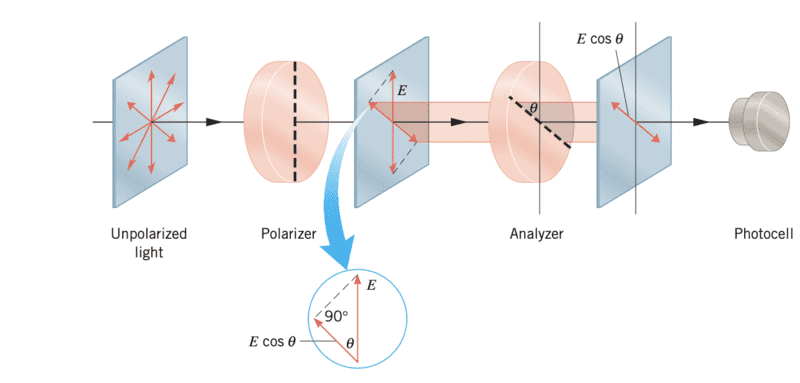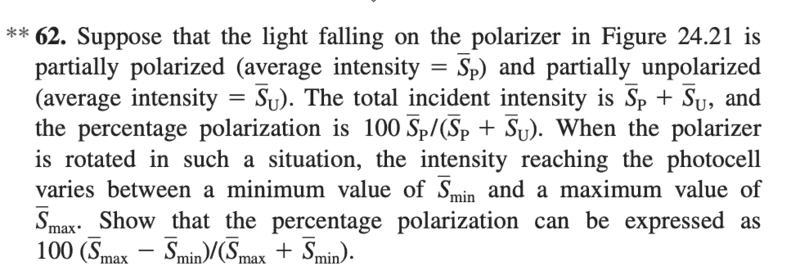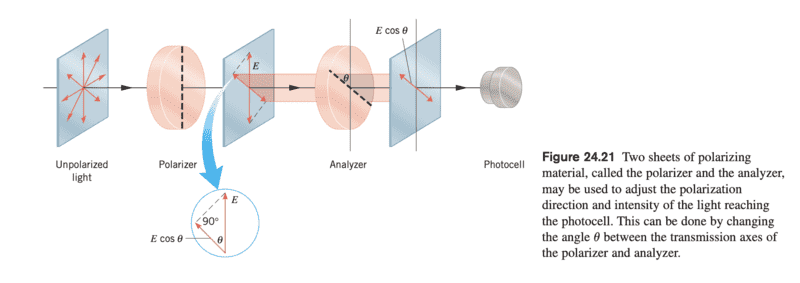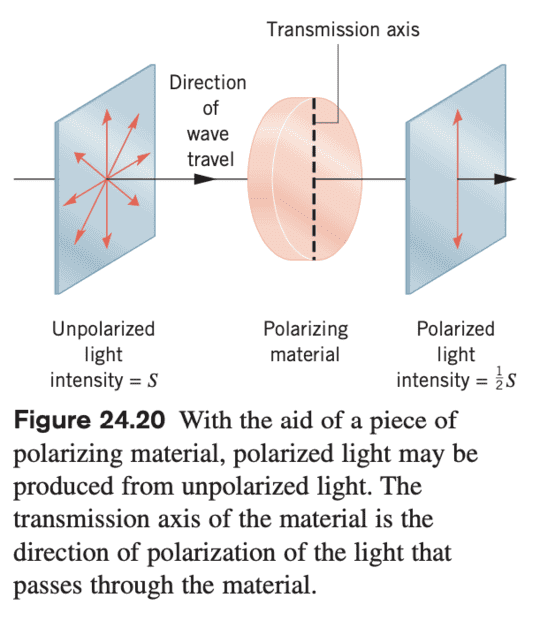# Effect of a polarizer/analyzer on partially polarized light

• FranzDiCoccio

#### FranzDiCoccio

Homework Statement
a partially polarized light is shone on a polarizer/analyzer system. Write the percentage polarization as a function of the maximum and minimum intensity emerging from the analyzer as the polarizer is rotated.
Relevant Equations
Malus law+intensity of unpolarized light emerging from a polarizer.
This is problem 62 in Cutnell & Johnson's Physics (9th edition):

Suppose that the light falling on the polarizer in the figure is partially polarized (average intensity $\bar S_P$) and partially upolarized (average intensity $\bar S_U$). The total incident intensity is $\bar S_P+ \bar S_U$ and the percentage polarization is $100 \bar S_P/(\bar S_P+ \bar S_U)$. When the polarizer is rotated in such a situation, the intensity reaching the photocell varies between a minimum value of $\bar S_{\min}$ and a maximum value of $\bar S_{\max}$.
Show that the percentage polarization can be expressed as $100 (\bar S_{\max}-\bar S_{\min})/(\bar S_{\max}+\bar S_{\min})$.I might not be understanding what the problem is asking, but I'd say that the proposed solution cannot be true.

The minimum intensity reaching the photocell as the polarizer is rotated is clearly zero, isnt'it? This correspond to the polarizer axis perpendicular to the analyzer axis. Whatever goes through the polarizer emerges polarized along its axis. When it subsequently hits the perpendicular analyzer it is completely blocked.
The maximum intensity happens when the polarizer axis is parallel to the electric field in the incident polarized component. In this situation the whole polarized component is transmitted, along with half of the unpolarized component. Whatever $\bar S_{\max}$ is, the solution proposed by the problem gives 100%, which does not make sense.

If I look at the intensity emerging from the polarizer only (and therefore not hitting the photocell yet) I get

$$\bar S_{\max} = \frac{1}{2} \bar S_U + \bar S_P \qquad \bar S_{\min} = \frac{1}{2} \bar S_U$$

In this case, I get the expected result, because $\bar S_{\max}+\bar S_{\min} =\bar S_P + \bar S_U$ and $\bar S_{\max}-\bar S_{\min} =\bar S_P$.

Another possibility is that the polarizer and the analyzer are rotated so that the angle between their transmission axes remains the same.
In this case

$$\bar S_{\max} = (\frac{1}{2} \bar S_U + \bar S_P) \cos^2 \theta \qquad \bar S_{\min} = \frac{1}{2} \bar S_U \cos^2 \theta$$

and the proposed solution also applies.

Maybe this is because I'm not a native English speaker, but it seems to me that the text of the problem states that the polarizer is rotated, while it should say that both the polarizer and the analyzer are rotated by the same angle, so that the angle between their axes stays constant.

Does this make sense?

I agree. If only the polariser, or only the analyzer, can be rotated then ##S_{min}## will always be zero (when the polarizer and the analyzer are ‘crossed’).

Maybe this is because I'm not a native English speaker, but it seems to me that the text of the problem states that the polarizer is rotated, while it should say that both the polarizer and the analyzer are rotated by the same angle, so that the angle between their axes stays constant.

Does this make sense?
Yes. I'd guess that is the intention of the (badly written) question.

•TSny
Hi Steve4Physics
thank you for your reply, and for your praise of my EnglishSometimes I worry I am not understanding something obvious because of the "language barrier". I thought that "polarizer" might mean the whole "polarized + analyzer" system, but I was not sure.
After all, I think that the standard use of such a system is that one can rotate the polarizer wrt the analyzer, to use Malus' law.
I guess blocking them at some fixed angle is just an artificial situation that was devised to create a new problem for the students to solve.

Could that be actually useful in some situation? I guess not, because one gets the same result by using only one polarizer...

Thanks again!

Sometimes I worry I am not understanding something obvious because of the "language barrier". I thought that "polarizer" might mean the whole "polarized + analyzer" system, but I was not sure.
In different contexts, I guess 'polarizer' could be used to mean an entire polarizer/analyzer system.

But in this question, 'polarizer' and 'analyzer' are clearly labelled in the diagram as two different objects. So the wording in the question appears to be wrong (or very unclear).

Could that be actually useful in some situation? I guess not, because one gets the same result by using only one polarizer...
I can't think of any uses. I'm no expert. But other members of the forum might be able to suggest uses.

I have a different interpretation.
Start with the system shown in the figure except that the incident light on the polarizer is partially polarized and not unpolarized as the figure says.
Let the intensity incident on the polarizer be ##\bar {S}_u+\bar{S}_p.## The question is what is the intensity emerging from the polarizer? I note that the polarized component of the incident light need not be along the axis of the polarizer. The emergent intensity will be $$\bar {S}_1=\frac{1}{2}\bar {S}_u+\bar{S}_p\cos^2\!\varphi$$ where ##\varphi~##is the angle between the initial polarization and the axis of the polarizer. Now all this light is polarized along the axis of the polarizer. If the angle between that axis and the axis of the analyzer is ##\theta##, then the intensity incident on the photocell is $$\bar S=S_1\cos^2\!\theta=\left(\frac{1}{2}\bar {S}_u+\bar{S}_p\cos^2\!\varphi\right)\cos^2\!\theta.$$This has a maximum and a minimum value depending on the value of ##\varphi##.

The problem is asking you to show that you can figure out the percentage polarization of the partially polarized light simply by measuring the maximum and minimum values at some arbitrary (but fixed) value of the analyzer relative to the polarizer.

It might be worth clarifying a few points to help the OP...

Let the intensity incident on the polarizer be ##\bar {S}_u+\bar{S}_p.## The question is what is the intensity emerging from the polarizer?
The question is about what is emerging from the analyzer, not the polarizer. (Unless 'polarizer' is used to mean the whole system - which was the language-issue originally confusing the OP.)

I note that the polarized component of the incident light need not be along the axis of the polarizer.
From what the OP wrote, I think they already knew this. Though it's hard to say for certain without seeing the detailed working.

The problem is asking you to show that you can figure out the percentage polarization of the partially polarized light simply by measuring the maximum and minimum values at some arbitrary (but fixed) value of the analyzer relative to the polarizer.
I think that's exactly what the OP's interpretation was when they wrote:
... the polarizer and the analyzer are rotated so that the angle between their transmission axes remains the same.

The question was badly written and in a foreign language for the OP.

•FranzDiCoccio
I have a different interpretation.
Hi kuruman,
It seems to me that, as far as the maths is concerned, we are saying the same thing.
You wrote the general form for the intensity reaching the photocell, while I wrote its maximum ($\cos(\phi)=1$) and minimum ($\cos(\phi)=0$) value, which are directly involved in the calculation.

However, one polarizer is enough to determine the percentage polarization. No need for the analyzer, right? See the "first guess" in my OP.

I sort of see what you mean, though. It is intesting to know.
If for some reason you do not have one single sheet of polarizing film, but instead you have two films that are glued together at a possibly unknown angle, you still can determine the percentage polarization.

Still, it looks like an "artificial" problem to me, and makes me want to change the wording in the original problem from Cutnell and Johnson even more.
I'd write something like "notice that even if you are forced to rotate both the analyzer and the polarizer so that the angle $\theta\neq 90°$ between them does not vary, you still can express the percentage polarization as....".

Last edited:
My interpretation in post #5 did not express clearly what I was in my mind and I can see why it is confusing to someone who cannot read my mind. When I wrote " ##\dots~##at some arbitrary (but fixed) value of the analyzer relative to the polarizer" I meant that the analyzer is fixed relative to some direction in the lab so that the polarizer has a continuously changing angle relative to it.

One does not have to rotate the polarizer and analyzer together for the formula to be valid and one is expected to show that. Although ##(\bar S_{\max}+\bar S_{\min})## and ##(\bar S_{\max}-\bar S_{\min})## depend on the direction of the analyzer, their ratio, and hence the percentage polarization, does not. That is the point of this problem, I think.

Hi kuruman,
not sure I follow now. My interpretation of the problem is that the angle $\theta$ between the analyzer and the polarizer is fixed, and the angle $\phi$ between the polarizer and the incident polarized light varies. This gives your second formula for the intensity at the photocell, which in turn gives my values for the maximum and minimum intensity at the photocell (with varying $\phi$).

I think that "… at some arbitrary (but fixed) value of the analyzer relative to the polarizer" means exactly that: the angle $\theta$ is fixed, and hence when you rotate the polarizer you rotate the analyzer as well, by the same angle.

Now I'm not sure I understand "the analyzer is fixed relative to some direction in the lab so that the polarizer has a continuously changing angle relative to it". Does that mean the same, or is this different from my interpretation above?

It seems to me that this means that the angle between the polarizer and the analyzer is not fixed.

But if that is the case, you're changing $\theta$, and the minimum intensity at the photocell is clearly 0.

Also, do you agree that one can determine the percentage polarization with one single polarizer (no analyzer needed)?

It seems to me that this means that the angle between the polarizer and the analyzer is not fixed.
That's what I mean. Let me explain some more.

Look at the drawing. There are dotted lines on the polarizer and the analyzer to indicate axis of each. The dotted line on the polarizer is along the vertical. The dotted line on the analyzer forms angle ##\theta## from the vertical. Now rotate the polarizer away from the vertical but keep the analyzer fixed at ##\theta## and find the maximum and minimum intensity at the photocell. Clearly, the number for the maximum and the number for the minimum recorded by the photocell will depend on the value of ##\theta##. The problem asks you to show that the formula works regardless of what ##\theta is.
Also, do you agree that one can determine the percentage polarization with one single polarizer (no analyzer needed)?
I agree with that, but that is not what the problem is asking. This is a homework problem that you have to solve as it is given to you which includes the analyzer. Think why you found it necessary to rotate the analyzer and polarizer together. I am saying that it is not necessary. You can leave the analyzer alone and rotate the polarizer only and still be able to determine the percentage polarization.

The minimum intensity reaching the photocell as the polarizer is rotated is clearly zero,
It does not specify the range through which the polarizer is rotated. But since the range could include at right angles to the analyzer, that doesn’t help.

Last edited:
That's what I mean. Let me explain some more.

Look at the drawing. There are dotted lines on the polarizer and the analyzer to indicate axis of each. The dotted line on the polarizer is along the vertical. The dotted line on the analyzer forms angle ##\theta## from the vertical. Now rotate the polarizer away from the vertical but keep the analyzer fixed at ##\theta## and find the maximum and minimum intensity at the photocell. Clearly, the number for the maximum and the number for the minimum recorded by the photocell will depend on the value of ##\theta##. The problem asks you to show that the formula works regardless of what ##\theta is.

No, I do not agree. Or better, as I mentioned, I think that the problem does not make sense this way.

If I can rotate the polarizer relative to the analyzer, I can get to the point where their axes are crossed. Nothing gets to the photocell in that situation, because whatever emerges from the polarizer is completely blocked by the analyzer. This means $\bar S_{\min} =0$. Now whatever $\bar S_{\max}$ is, $(\bar S_{\max}-\bar S_{\min})/(\bar S_{\max}+\bar S_{\min}) 100= 100$. But that's not really true, is it? The incident light is not 100% polarized.

I agree with that, but that is not what the problem is asking. This is a homework problem that you have to solve as it is given to you which includes the analyzer. Think why you found it necessary to rotate the analyzer and polarizer together. I am saying that it is not necessary. You can leave the analyzer alone and rotate the polarizer only and still be able to determine the percentage polarization.

Of course. My observation was in response to the practical "value" of the situation portrayed in the problem.
I think that the exercise makes sense only if $\theta$ is fixed, and this is interesting if at the practical level the only thing at my disposal to determine the percentage polarization is two films glued at an angle.
However I do not really need a second polarizer to determine that.

It does not specify the range through which the polarizer is rotated.

Wait, now I cannot rotate it all the way?

So what you're saying is that I rotate between some angle and some other angle, which do not necessarily correspond to the absolute maximum and absolute minimum of the intensity?

I choose a value for $\theta$ look at the intensity, then change $\theta$ by rotating the polarizer and look at the intensity again. One of the intensities is probably less than the other and I call those $\bar S_{\min}$ and $\bar S_{\max}$.
Now you're saying that irrespective of the choice of the angles, one is able to find the percentage polarization.

I see.. I have to think about it. But even if this works, I still think that the problem is not clearly phrased.
The minimum intensity on the photocell as $\theta$ is varied is zero.

Wait, now I cannot rotate it all the way?

So what you're saying is that I rotate between some angle and some other angle, which do not necessarily correspond to the absolute maximum and absolute minimum of the intensity?

I choose a value for $\theta$ look at the intensity, then change $\theta$ by rotating the polarizer and look at the intensity again. One of the intensities is probably less than the other and I call those $\bar S_{\min}$ and $\bar S_{\max}$.
Now you're saying that irrespective of the choice of the angles, one is able to find the percentage polarization.

I see.. I have to think about it. But even if this works, I still think that the problem is not clearly phrased.
The minimum intensity on the photocell as $\theta$ is varied is zero.

Sorry I keep confusing the angles... Kuruman says that $\theta$ (i.e. the position of the analyzer) is fixed, and the polarizer is rotated.
But rotating the polarizer does change the angle between the analyzer and the polarizer, whatever we may call that. Also it changes the angle between the polarization direction of the polarized component and the transmission axis of the polarizer (kuruman's $\varphi$). These changes are not independent.

Thank you all for your help and patience.

These changes are not independent.
Yes. The angle between the initial polarization ##\bar S_p## and the analyzer is fixed.

The only way I can make sense of it is if the unpolarised light is bypassing the polariser and falling directly on the analyser. Then I get the given answer.

Yes. The angle between the initial polarization ##\bar S_p## and the analyzer is fixed.
How does that help? The rotation range of the polariser could include being at right angles to the analyser, at which point the photocell gets nothing so ##\bar S_{min}=0##. As @FranzDiCoccio notes, the formula then gives ##\bar S_U=0##, regardless of its actual value.

•FranzDiCoccio
It does not specify the range through which the polarizer is rotated. But since the range could include at right angles to the analyzer, that doesn’t help.

Oh ok, I see the edited message now. This is the problem which had me start this topic.
By the way, I'm realizing just now that I was not very precise in my OP. The problem is n° 62 in chapter 24 (page 767 in my version of Cutnell and Johnson's "Physics, 9th edition").

The only way I can make sense of it is if the unpolarised light is bypassing the polariser and falling directly on the analyser. Then I get the given answer.

This is what I initialy thought as well.
There is actually one other way to get the proposed result, that is assuming that the angle between the polarizer and the analyzer is fixed, so that when you rotate the former you also rotate the latter by the same angle.
See my OP, which I think agrees with kuruman's first comment: my formulas for the maximum and minimum intensity correspond to $\varphi = k \pi$ and $\varphi = (2k-1) \pi/2$ in their second formula.

I would agree with OP's original statement that the analyzer is not needed or can be rotated together with the polarizer for this problem to make sense. However, I wonder if the accompanying figure belongs to this problem. The figure shows on the far left the input as "unpolarized light." This directly contradicts the text that mentions "partially polarized light." It does not match the text, therefore it is undoubtedly the wrong figure. If the correct figure exists somewhere out there, how sure are we that it shows an analyzer? Note that there is no mention of an analyzer in the text. The lack of an analyzer in the figure would put everything in its place.

•FranzDiCoccio, haruspex and berkeman
I would agree with OP's original statement that the analyzer is not needed or can be rotated together with the polarizer for this problem to make sense. However, I wonder if the accompanying figure belongs to this problem. The figure shows on the far left the input as "unpolarized light." This directly contradicts the text that mentions "partially polarized light." It does not match the text, therefore it is undoubtedly the wrong figure. If the correct figure exists somewhere out there, how sure are we that it shows an analyzer? Note that there is no mention of an analyzer in the text. The lack of an analyzer in the figure would put everything in its place.
I think you have it.
I took the blue text in post #1 as the continuation of the homework statement "a partially polarized light is shone on a polarizer/analyzer system", but if the latter was merely inferred from the diagram then a rogue diagram explains all.

I wonder if the same diagram appears, correctly, in another question.

•FranzDiCoccio
I wonder if the same diagram appears, correctly, in another question.
Maybe @FranzDiCoccio who has access to the textbook can shed some light into this matter.

Hi,
i copied the text basically verbatim. I just did not copy the reference to the figure.
The figure I included in my OP is indeed figure 24.21. This is at page 755, in the theory part.
The text of the exercise specifically refers to that figure, as far as the configuration of the analyzer-polarizer system is concerned.

Below I'm attaching the original text of problem 62 (page 767) and the complete figure 24.21.
Now I think I see what you mean... It might be a misnumbering of the relevant figure.
Probably the author of the problem did not mean figure 24.21, but rather figure 24.20, which I'm also attaching below.
Now that makes sense...

I should have thought of that. The fact that the term "polarizer" is introduced in figure 24.21 is slightly misleading, though. Also maybe I would call the sheet of polarizing film used for in problem 62 an analyzer rather than a polarizer. But I think I'm nitpicking.#### Attachments

Yes, a typo almost explains it. But note the text refers to a photocell, and there is none in 24.20. So I'd say the author tried to get away without drawing another diagram.

Below I'm attaching the original text of problem 62 (page 767) and the complete figure 24.21.
Now I think I see what you mean... It might be a misnumbering of the relevant figure.
Probably the author of the problem did not mean figure 24.21, but rather figure 24.20, which I'm also attaching below.
Now that makes sense...
I think you are correct and nobody picked up the error. The 9th edition was out in January 2012. It would be informative to see if the most recent edition has this problem and in a corrected form.

Hi all,
I discovered that the book comes with an Instructor's Manual. I asked around and someone provided the "official" solution to problem 62.
The "reasoning and solution" seems to indicate that there was no error in referring to fig. 24.21. The reasoning never explicitly mentions this, but kind of confirms that the polarizer and analyzer are rotated together by the same amount. Indeed the calculations are exactly as in the second scenario in my OP, in that both the maximum and minimum intensity are proportional to $\cos^2 \theta$.
If there was only one polarizing sheet, like in fig. 24.20, there would not be such a factor (like in my first scenario).
Also, as @haruspex observes, the text of the problem explicitly mentions a photocell, which is present in fig 24.21 and absent in fig. 24.20.

I'd be curious to see whether more recent editions clarify that the polarizer and analyzer must be rotated together. I do not think that this is obvious from what is explained in the book: for instance, the caption of fig. 24.21 explicitly says that $\theta$ changes. This is confusing for a student, I think.

After all this seems to be one of those problem that is immediately clear only to people whose job is inventing textbook problems.

•Rive